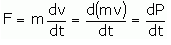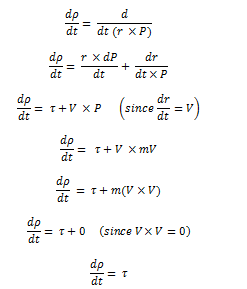The quantity of rotational motion in a body is called its angular momentum. Thus angular momentum plays the same role in rotational motion as played by linear momentum in translational motion.

Mathematically, angular momentum is the cross-product of the position vector and the linear momentum, both measured in an inertial frame of reference.

ρ = r × P

The magnitude of the angular momentum vector is

ρ = r P sinθ (magnitude)

ρ = r m V sinθ (since P = m V)

where, V is linear speed, θ is the angle between r and P

θ= 90° in circular motion (special case)

The direction of the angular momentum can be determined by the Right-Hand Rule.

Also ρ = r m (r ω) sinθ

ρ = m (r2 ω) sinθ

Units of Angular Momentum

The units of angular momentum in S.I system are kgm2/s or Js.

1. ρ = r m V sinθ

= m × kg × m/s

= kg.m2/s

1. ρ = r P sinθ

= m × Ns

= (Nm) × s

= J.s

Dimensions of Angular Momentum

[ρ] = [r][P]

= [r] [m] [V]

= L.M.L/T

= L2 M T-1

Relation between Torque and Angular Momentum

Or

Prove that the rate of change of angular momentum is equal to the external torque acting on the body.

Proof:- We know that rate of change of linear momentum is equal to the applied force.

F = maWhere P = mv is a linear momentum of the particle.

Taking the vector product of r with both sides, we get

But r × F = , Therefore, we have

= r × (since τ = r × P)

Now, according to the definition of angular momentum

ρ = r × P

Taking derivative w.r.t time, we getOr, Rate of change of Angular Momentum = External Torque (Proved).

## Conservation of Angular Momentum

According to the law of conservation of angular momentum, if the external torque (couple) acting on a system is zero, the total angular momentum of a rigid body or a system of particles is conserved.

If the moment of inertia of the body changes from I1 to I2 due to the change of the distribution of mass of the body, then angular velocity of the body changes from ω1 to ω2, such that

If τext 0, then L = I(ω) = constant ⇒ I1ω1 = I2ω2